# Malware analysis: part 8. Yara rule example for MurmurHash2. MurmurHash2 in Conti ransomware

Hello, cybersecurity enthusiasts and white hackers!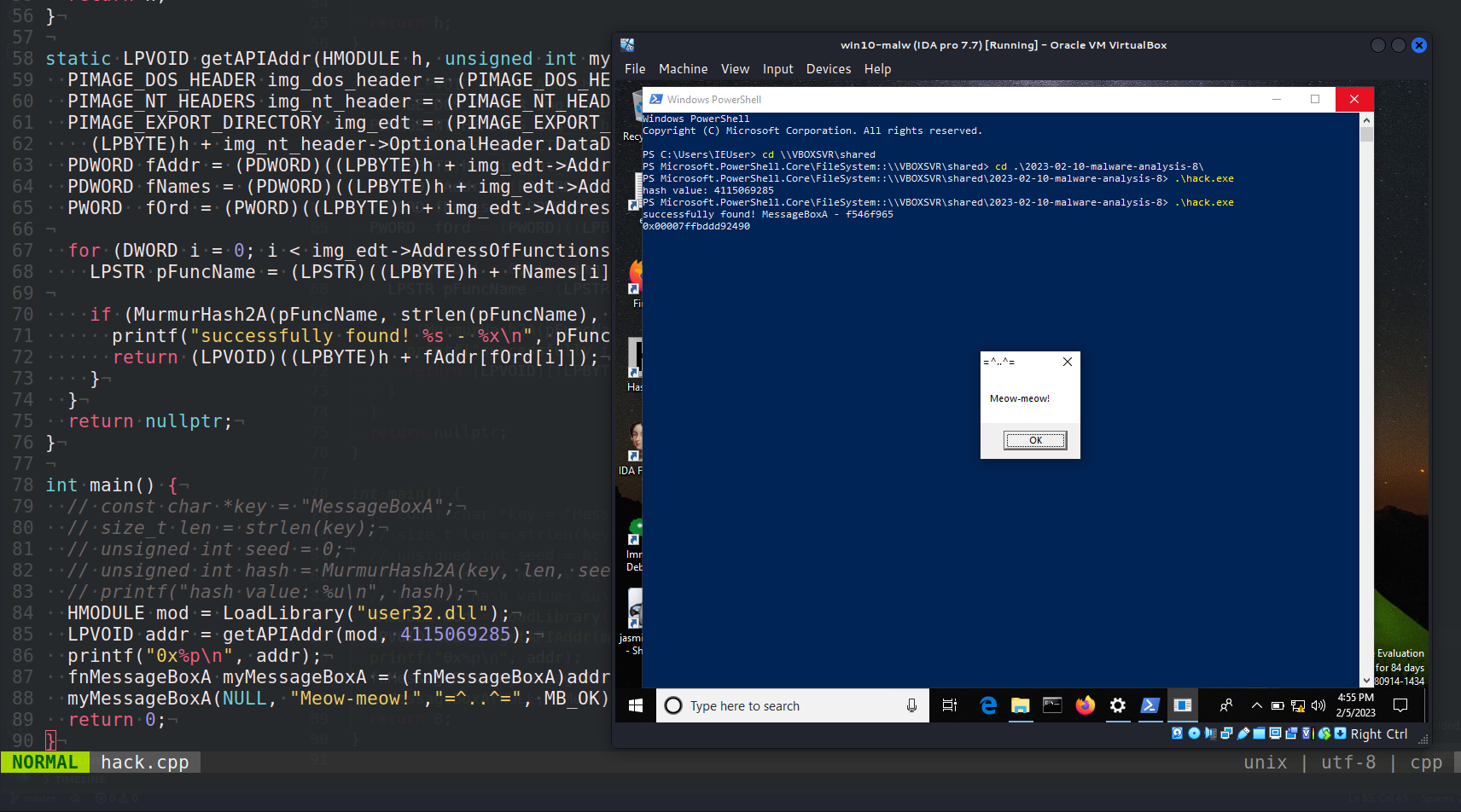This post is the result of my own research on Yara rule for `Murmurhash2` hashing. How to use it for malware analysis in practice.

### MurmurHash

MurmurHash2A is a non-cryptographic hash function optimized for performance and speed. It divides the input data into `4-byte` blocks, applies bitwise operations and XORs to each block, and then uses a finalizer to produce the final hash result.

Here’s a high-level overview of the algorithm:

1. divide the input data into `4-byte` blocks.
2. initialize a seed value, which is used to influence the final hash value.
3. for each block, perform bitwise operations such as XORs, multiplications, and bit rotations to produce a new intermediate value, the calculation of the intermediate value includes the constant value `0x5bd1e995`.
4. XOR the intermediate value with the seed.
5. Repeat steps 3 and 4 for each block.
6. Use a finalizer to mix the intermediate value and produce the final hash value.

So as you can see, MurmurHash2A with a constant value of `0x5bd1e995` is a variation of the MurmurHash2A algorithm. The constant value is incorporated into the calculation.

The MurmurHash2 algorithm was created by Austin Appleby.

### practical example

This algorithm is also often used for hashing function names.
For example, if you look at the source code of the Conti ransomware leak, you can see `Murmurhash2A` function.

It might look something like this (`hack.cpp`):

``````/*
* hack.cpp - hashing Win32API functions via MurmurHash2A. C++ implementation
* @cocomelonc
* https://cocomelonc.github.io/malware/2023/02/10/malware-analysis-8.html
*/
#include <stdint.h>
#include <stdlib.h>
#include <stdio.h>
#include <string.h>
#include <windows.h>

typedef UINT(CALLBACK* fnMessageBoxA)(
HWND   hWnd,
LPCSTR lpText,
LPCSTR lpCaption,
UINT   uType
);

// MurmurHash is a non-cryptographic hash function and was written by Austin Appleby.
unsigned int MurmurHash2A(const void *key, size_t len, unsigned int seed) {
const unsigned int m = 0x5bd1e995;
const int r = 24;
unsigned int h = seed ^ len;

const unsigned char *data = (const unsigned char *)key;

while (len >= 4) {
unsigned int k = *(unsigned int *)data;

k *= m;
k ^= k >> r;
k *= m;

h *= m;
h ^= k;

data += 4;
len -= 4;
}

switch (len) {
case 3:
h ^= data << 16;
case 2:
h ^= data << 8;
case 1:
h ^= data;
h *= m;
};

h ^= h >> 13;
h *= m;
h ^= h >> 15;

return h;
}

static LPVOID getAPIAddr(HMODULE h, unsigned int myHash) {
PIMAGE_EXPORT_DIRECTORY img_edt = (PIMAGE_EXPORT_DIRECTORY)(
PDWORD fNames = (PDWORD)((LPBYTE)h + img_edt->AddressOfNames);
PWORD  fOrd = (PWORD)((LPBYTE)h + img_edt->AddressOfNameOrdinals);

for (DWORD i = 0; i < img_edt->AddressOfFunctions; i++) {
LPSTR pFuncName = (LPSTR)((LPBYTE)h + fNames[i]);

if (MurmurHash2A(pFuncName, strlen(pFuncName), 0) == myHash) {
printf("successfully found! %s - %x\n", pFuncName, myHash);
}
}
return nullptr;
}

int main() {
// const char *key = "MessageBoxA";
// size_t len = strlen(key);
// unsigned int seed = 0;
// unsigned int hash = MurmurHash2A(key, len, seed);
// printf("hash value: %u\n", hash);
myMessageBoxA(NULL, "Meow-meow!","=^..^=", MB_OK);
return 0;
}
``````

As you can see, as usually I used `MessageBoxA` WinAPI function for experiment.

### demo

Let’s go see using MurmurHash for hashing function names in action.

Compile our “malware”:

``````x86_64-w64-mingw32-g++ -O2 hack.cpp -o hack.exe -I/usr/share/mingw-w64/include/ -s -ffunction-sections -fdata-sections -Wno-write-strings -fno-exceptions -fmerge-all-constants -static-libstdc++ -static-libgcc -fpermissive
``````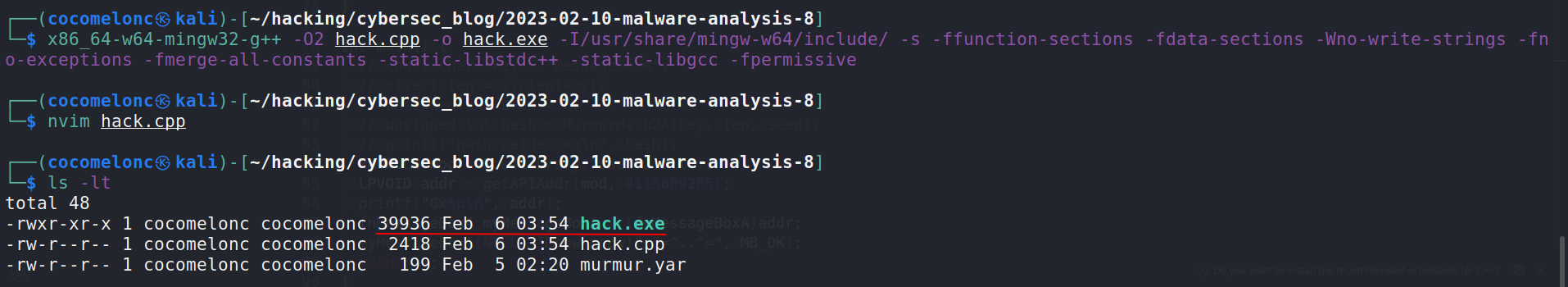Run it at the victim’s machine:

``````.\hack.exe
``````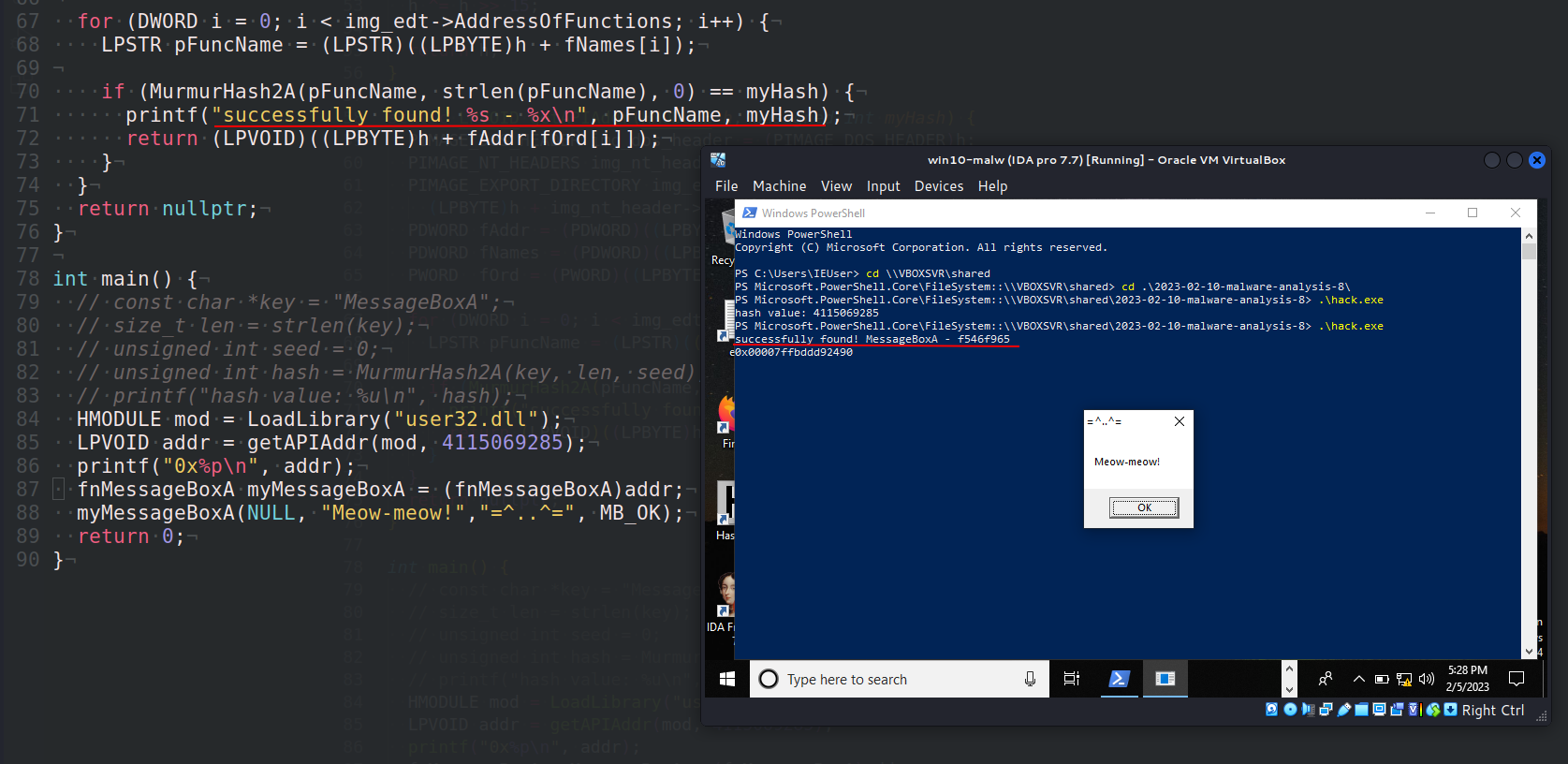As you can see, everything is worked perfectly! =^..^=

Let’s go to upload our “malware” to VirusTotal:So, 4 of 70 AV engines detect our file as malicious.

https://www.virustotal.com/gui/file/3975c386fedf96000f97e20675f76a23407b41f7e9909eaf22fbf90cf03fa211/details

### yara rule

In the simplest implementation, the Yara rule will look like this:

``````rule murmurhash2_rule {
meta:
author = "cocomelonc"
description = "example rule using MurmurHash2A with constant 0x5bd1e995"
strings:
\$hash = { 95 e9 d1 5b }
condition:
\$hash
}
``````

As you can see, we just add algorithm’s constant for identity:

``````hexdump -C ./hack.exe | grep "95 e9 d1 5b"
``````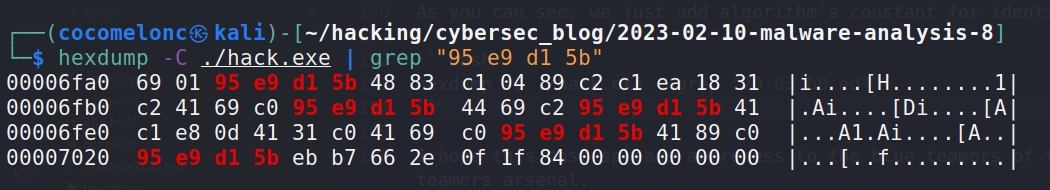Run it:

``````yarar -w ./murmur.yar -r ./
``````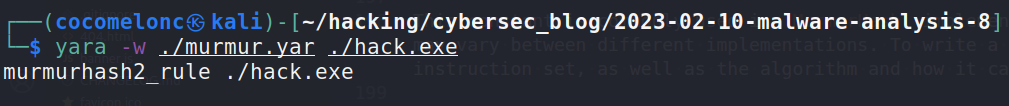This constant is commonly used in `MurmurHash` implementations, but the specific constants, instructions, and their ordering may vary between different implementations. To write a good YARA rule, you need to know a lot about the architecture and instruction set, as well as the algorithm and how it can change.

So, what are the advantages of the `MurmurHash2` algorithm? `MurmurHash2` is fast and efficient and is suitable for hashing large amounts of data in real-time applications. `MurmurHash2` has good collision resistance, which means that it generates unique hash values for different input data, making it suitable for use in hash tables and other data structures where hash collisions need to be avoided. `MurmurHash2` is a cross-platform algorithm that can be easily implemented in different programming languages and environments. For example, python implementation:

``````def murmurhash2(key: bytes, seed: int) -> int:
m = 0x5bd1e995
r = 24
h = seed ^ len(key)
data = bytearray(key) + b'\x00' * (4 - (len(key) & 3))
data = memoryview(data).cast("I")
for i in range(len(data) // 4):
k = data[i]
k *= m
k ^= k >> r
k *= m
h *= m
h ^= k
h ^= h >> 13
h *= m
h ^= h >> 15
return h

h = murmurhash2(b"meow-meow", 0)
print ("%x" % h)
print ("%d" % h)
``````

Note that `MurmurHash2` is not designed to be a cryptographic hash function and should not be used for secure applications that require cryptographic-strength hash functions, such as password storage or digital signatures.

This hash is used by Conti ransomware and `Win32/Potao` malware family at the wild.

I hope this post spreads awareness to the blue teamers of this interesting hashing technique, and adds a weapon to the red teamers arsenal.

This is a practical case for educational purposes only.

Thanks for your time happy hacking and good bye!
PS. All drawings and screenshots are mine

Tags:

Categories:

Updated: